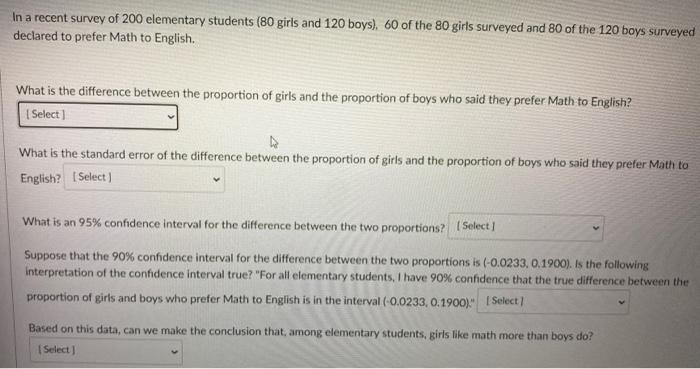# Question In a recent survey of 200 elementary students (80 girls and 120 boys) 60 of the 80 girls surveyed and 80 of the 120 boys surveyed declared to prefer Math to English. What is the difference between the proportion of girls and the proportion of boys who said they prefer Math to English? Select] What is the standard error of the difference between the proportion of girls and the proportion of boys who said they prefer Math to English? Select) What is an 95% confidence interval for the difference between the two proportions? Select Suppose that the 90% confidence interval for the difference between the two proportions is (-0.0233,0,1900). Is the following Interpretation of the confidence interval true? "For all elementary students, I have 90% confidence that the true difference between the proportion of girls and boys who prefer Math to English is in the interval (-0.0233, 0.1900). Select Based on this data, can we make the conclusion that, among elementary students, girls like math more than boys do? Select)IIRBKR The Asker · Probability and StatisticsTranscribed Image Text: In a recent survey of 200 elementary students (80 girls and 120 boys) 60 of the 80 girls surveyed and 80 of the 120 boys surveyed declared to prefer Math to English. What is the difference between the proportion of girls and the proportion of boys who said they prefer Math to English? Select] What is the standard error of the difference between the proportion of girls and the proportion of boys who said they prefer Math to English? Select) What is an 95% confidence interval for the difference between the two proportions? Select Suppose that the 90% confidence interval for the difference between the two proportions is (-0.0233,0,1900). Is the following Interpretation of the confidence interval true? "For all elementary students, I have 90% confidence that the true difference between the proportion of girls and boys who prefer Math to English is in the interval (-0.0233, 0.1900). Select Based on this data, can we make the conclusion that, among elementary students, girls like math more than boys do? Select)
More
Transcribed Image Text: In a recent survey of 200 elementary students (80 girls and 120 boys) 60 of the 80 girls surveyed and 80 of the 120 boys surveyed declared to prefer Math to English. What is the difference between the proportion of girls and the proportion of boys who said they prefer Math to English? Select] What is the standard error of the difference between the proportion of girls and the proportion of boys who said they prefer Math to English? Select) What is an 95% confidence interval for the difference between the two proportions? Select Suppose that the 90% confidence interval for the difference between the two proportions is (-0.0233,0,1900). Is the following Interpretation of the confidence interval true? "For all elementary students, I have 90% confidence that the true difference between the proportion of girls and boys who prefer Math to English is in the interval (-0.0233, 0.1900). Select Based on this data, can we make the conclusion that, among elementary students, girls like math more than boys do? Select)